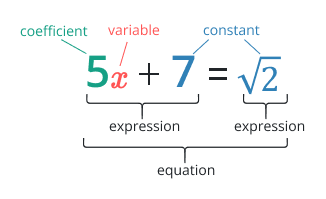# Equation Solver

Enter the equation and hit calculate button to solve the equation using this calculatorGive Us Feedback

## Equation Solver

Equation Solver is a tool used to solve polynomial equations of any order, such as linear or quadratic equations. It employs methods like adding or subtracting the same variable term and manipulating the equation to find the value(s) of the variable that satisfies the equation.

## What is meant by equation and expression?

### Equation

It states that the two expressions are equal is known as an equation. Simply say that it is a combination of two expressions that are distributive by the equal sign. Equations are useful for clarifying the connection between various variables and a constant. It plays a crucial role in solving many mathematical expressions and problems.

i.e., 2x + 5 = 10 + x is an equation with the variable “x”.

### Expression

It is a mixture of numbers, variables, and mathematical operations (such as addition and subtraction) while not an equal sign. Expressions can be as simple as a single number or variable or more complex, involving multiple terms and operations.

i.e., 3x + 7 is the expression with the variable “x”.## How to solve the equation?

Example 1:

4x2 + 2x2 - 4 = 0 solve the equation.

Solution

Step 1: Collect and add the like terms.

(4x2 + 2x2) - 4 = 0

Step 2: Add the polynomial terms together.

(6x2) - 4 = 0

Step 3: Add the constant term from both sides.

6x2 - 4 + 4 = 0 + 4

Step 4: Simplify the left and right-hand side.

6x2 = 4

Step 5: To find the value of “x” divide by “6” on both sides and take square root.

6x2 /6 = 4/6

x2 = 2/3

x2 = √2/3

x = ∓ 0.8165

Example 2:

2x + x + 9 = 0 solve the equation.

Solution

Step 1: Collect and add the like terms.

(2x + x) + 9 = 0

Step 2: Add the polynomial terms together.

(3x) + 9 = 0

Step 3: Subtract the constant term from both sides.

3x + 9 − 9 = 0 – 9

Step 4: Simplify the left and right-hand sides.

3x = −9

Step 5: To find the value of “x” divide by “3” on both sides and simplify.

3x/3 = − 9/3

x = − 3

### Math Tools